# NCERT Solutions for Class 6 Maths Chapter 9 Data Handling

NCERT Solutions for Class 6 Maths Chapter 9 Data Handling

### NCERT Solutions for Class 6 Maths Chapter 9 Data Handling Ex 9.1

NCERT Solutions for Class 6 Maths Chapter 9 Data Handling Ex 9.1

Exercise 9.1

Ex 9.1 Class 6 Maths Question 1.
In a Mathematics test, the following marks were obtained by 40 students. Arrange these marks in a table using tally marks.(a) Find how many students obtained marks equal to or more than 7.
(b) How many students obtained marks below 4?
Solution:
From the given data, we have the following table.(a) Number of students who obtained marks equal to or more than 7 = 5 + 4 + 3 = 12
(b) Number of students who obtained marks below 4 = 2 + 3 + 3 = 8.

Ex 9.1 Class 6 Maths Question 2.
Following is the choice of sweets of 30 students of Class VI.
Arrange the names of sweets in a table using tally marks.
Which sweet is preferred by most of the students?
Solution:
(a) We have the following table: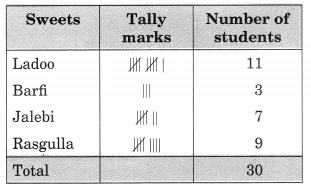(b) Ladoo is preferred by most of the students, i.e., 11 students.

Ex 9.1 Class 6 Maths Question 3.
Catherine threw a dice 40 times and noted the number appearing each time as shown below:Make a table and enter the data using tally marks. Find the number that appeared.
(а) The minimum number of times
(b) The maximum number of times
(c) Find those numbers that appear an equal number of times.
Solution:
We have the following table:From the above table, we get
(a) The number 4 appeared 4 times which is the minimum.
(b) The number 5 appeared 11 times which is the maximum.
(c) The number 1 and 6 appear for the same number of times, i.e., 7.

Ex 9.1 Class 6 Maths Question 4.
Following pictograph shows the number of tractors in five villages.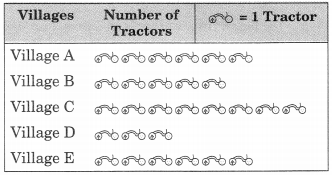Observe the pictograph and answer the following questions.
(a) Which village has the minimum number of tractors?
(b) Which village has the maximum number of tractors?
(c) How many more tractors village C has as compared to village B?
(d) What is the total number of tractors in all. the five villages?
Solution:
From the given pictograph, we have
(а) Village D has the minimum number of tractors, i.e., 3.
(б) Village C has the maximum number of tractors, i.e., 8.
(c) Village C has 3 tractors more than that of the village B.
(d) Total number of tractors in all the villages is 28.

Ex 9.1 Class 6 Maths Question 5.
The number of girl students in each class of a co-educational middle school is depicted by the pictograph:Observe this pictograph and answer the following questions:
(a) Which class has the minimum number of girl students?

(b) Is the number of girls in Class VI less than the number of girls in Class V?
(c) How many girls are there in Class VII?
Solution:Ex 9.1 Class 6 Maths Question 6.
The sale of electric bulbs on different days of a week is shown below: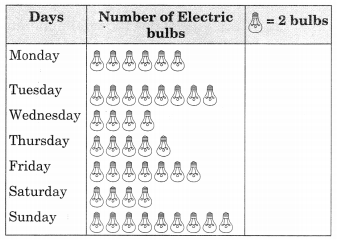Observe the pictograph and answer the following questions:
(a) How many bulbs were sold on Friday?
(b) On which day were the maximum number of bulbs sold?
(c) On which of the days same number of bulbs were sold?

(d) On which of the days minimum number of bulbs were sold?

(e) If one big carton can hold 9 bulbs. How many cartons were needed in the given week?
Solution:
(a) Number of bulbs sold on Friday = 7 x 2 = 14
(b) On Sunday, the number of bulbs sold = 9 x 2 = 18 which is maximum in number.
(c) On Wednesday and Saturday, the same number of bulbs were sold, i.e., 4 x 2 = 8
(d) The minimum number of bulbs were sold on Wednesday and Saturday, i.e., 4 x 2 = 8
(e) Total number of bulbs sold in a week = 43
Number of cartons needed 5Ex 9.1 Class 6 Maths Question 7.
In a village six fruit merchants sold the following number of fruit baskets in a particular season:Observe this pictograph and answer the following questions:
(a) Which merchant sold the maximum number of baskets?
(b) How many fruit baskets were sold by Anwar?
(c) The merchants who have sold 600 or more number of baskets are planning to buy a godown for the next season. Can you name them?
Solution:
(a) Martin sold the maximum number of fruit baskets, i.e.,(c) Number of fruit baskets sold by Anwar is 7 x 100 = 700.
(c) Anwar, Martin and Ranjit Singh have sold 600 or more fruit baskets and planning to buy a godown.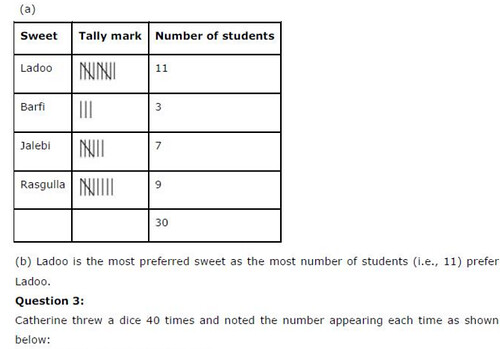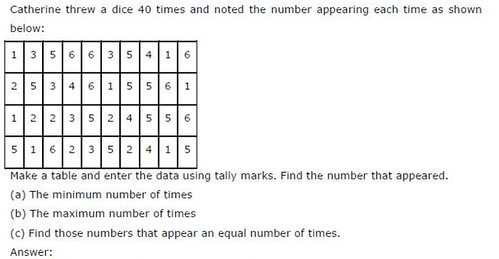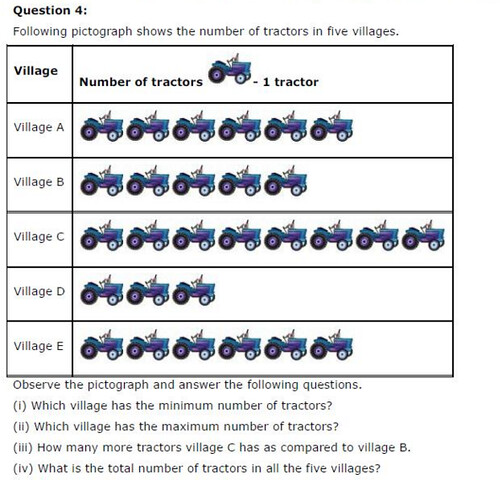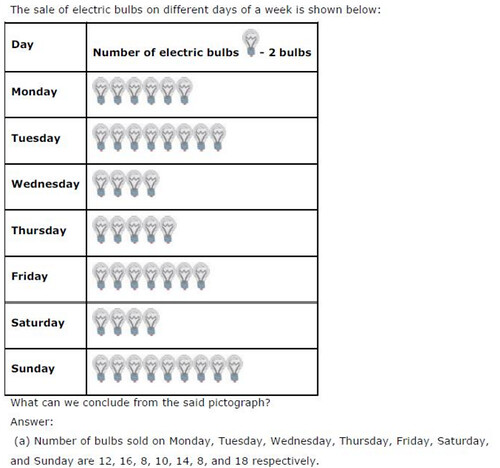## SabDekho

The Complete Educational Website